Author: Oscar Cronquist Article last updated on June 11, 2018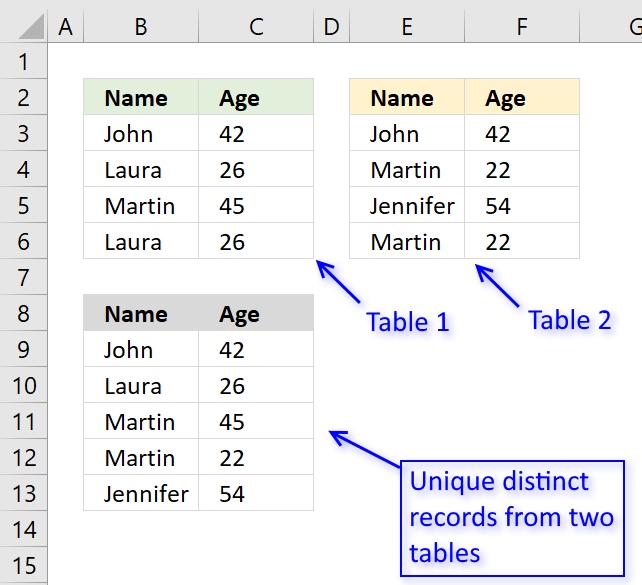The picture above shows an array formula in cell B9:C13 that extracts unique distinct records from two tables in cell range B3:C6 and E3:F6.

If a record exists in both tables only one record is returned by the formula. If a record exists multiple times in one table only one record is returned by the formula.

Example, John 42 exists in both tables, however, the formula returns only one instance of John 42.

Laura 26 exists multiple times but only in the first table, the formula returns only one record of Laura 26.

Array formula in cell B9:

=IFNA(INDEX(\$B\$3:\$C\$6, MATCH(0, COUNTIFS(\$B\$8:B8, \$B\$3:\$B\$6, \$C\$8:C8, \$C\$3:\$C\$6), 0),COLUMNS(\$A\$1:A1)), INDEX(\$E\$3:\$F\$6,MATCH(0, COUNTIFS(\$B\$8:B8, \$E\$3:\$E\$6, \$C\$8:C8, \$F\$3:\$F\$6), 0), COLUMNS(\$A\$1:A1)))

To enter an array formula, type the formula in cell B9 then press and hold CTRL + SHIFT simultaneously, now press Enter once. Release all keys.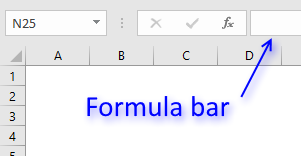The formula bar now shows the formula with a beginning and ending curly bracket telling you that you entered the formula successfully.

Don't enter the curly brackets yourself, they appear automatically.

### What is a unique distinct record?

Unique distinct records are all records except duplicates merged into one distinct value.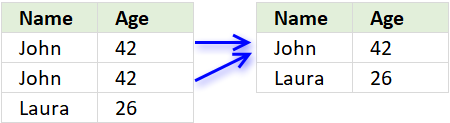In other words, duplicate records are removed.

### Explaining the formula in cell B9

Use the "Evaluate Formula" tool to examine the calculation steps in greater detail.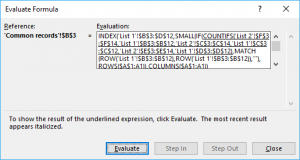Go to tab "Formula" on the ribbon, press with left mouse button on "Evaluate Formula" button to start the tool.

Then press with left mouse button on the "Evaluate" button to see the next step in the calculation, this will make it easier to understand how the formula works.

#### Step 1 - Count previous records against table 1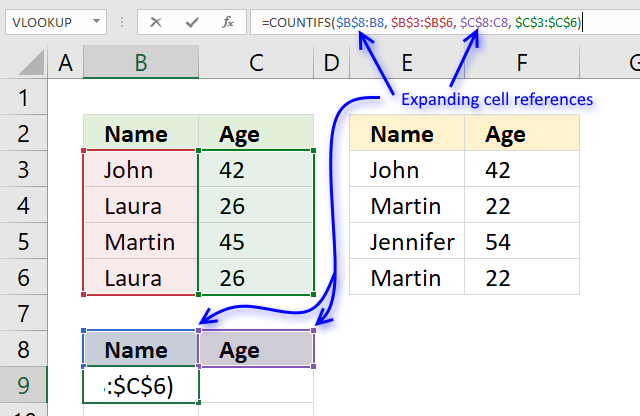The COUNTIFS function allows you to count how many times a record exists in a table.

The previous values in cell B9 are the values in B8 and C8.

The first argument \$B\$8:B8 in the COUNTIFS function has both absolute and relative cell references, this allows the formula to automatically expand when you copy it and paste to cells below.

"Name" and "Age" is not found in cell range B3:C6 so the COUNTIFS function returns 0 (zero) for each record.

COUNTIFS(\$B\$8:B8, \$B\$3:\$B\$6, \$C\$8:C8, \$C\$3:\$C\$6)

becomes

=COUNTIFS("Name", {"John";"Laura";"Martin";"Laura"}, "Age", {42;26;45;26})

and returns {0;0;0;0}. There are four records and the function returns an array containing 4 values (zeros).

#### Step 2 - Find the first instance of 0 (zero) in the array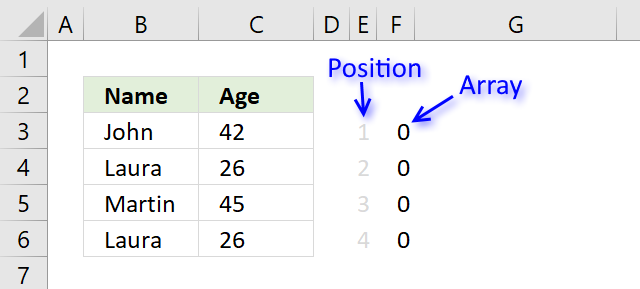The MATCH function allows you to identify which record to return next.

MATCH(0, COUNTIFS(\$B\$8:B8, \$B\$3:\$B\$6, \$C\$8:C8, \$C\$3:\$C\$6), 0)

becomes

MATCH(0, {0;0;0;0}, 0)

and returns 1. The first instance of 0 (zero) is found in position 1 in the array.

#### Step 3 - Return value from a record

The INDEX function lets get a specific value using a row and column number.

The MATCH function calculates the row number we need to get the correct value, however, the COLUMNS function keeps track of which value in the record to get.

The COLUMNS function calculates the number of columns in a cell reference, the cell reference used here \$A\$1:A1 is also expanding when the formula is copied to other cells.

COLUMNS(\$A\$1:A1) returns 1.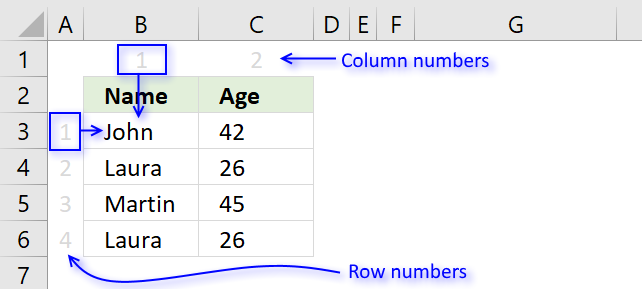INDEX(\$B\$3:\$C\$6, MATCH(0, COUNTIFS(\$B\$8:B8, \$B\$3:\$B\$6, \$C\$8:C8, \$C\$3:\$C\$6), 0),COLUMNS(\$A\$1:A1))

becomes

INDEX(\$B\$3:\$C\$6, 1, COLUMNS(\$A\$1:A1))

becomes

INDEX(\$B\$3:\$C\$6, 1, 1) and returns John in cell B9.

#### Step 4 - IFNA function points the calculation in a new direction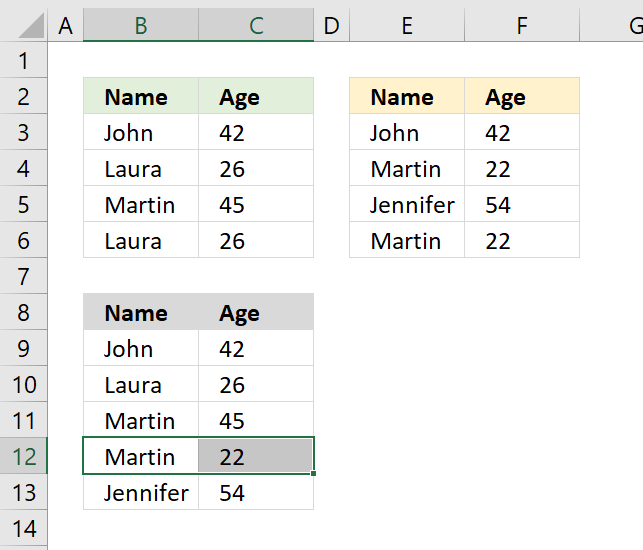Step 1 to 3 explains how the formula extracts unique distinct records from the first table.

The first part of the formula returns a #N/A error when there are no records left in table 1 to extract.

The IFNA function points the calculation to part2 when the error occurs.

=IFNA(part1, part2)

The second part of the formula does the exact same thing as the first part except that the cell references this time points to the second table.

The picture above shows that the two last records are extracted from the second table.

### Get Excel *.xlsx file

Extract unique distinct records from two data sets.xlsx